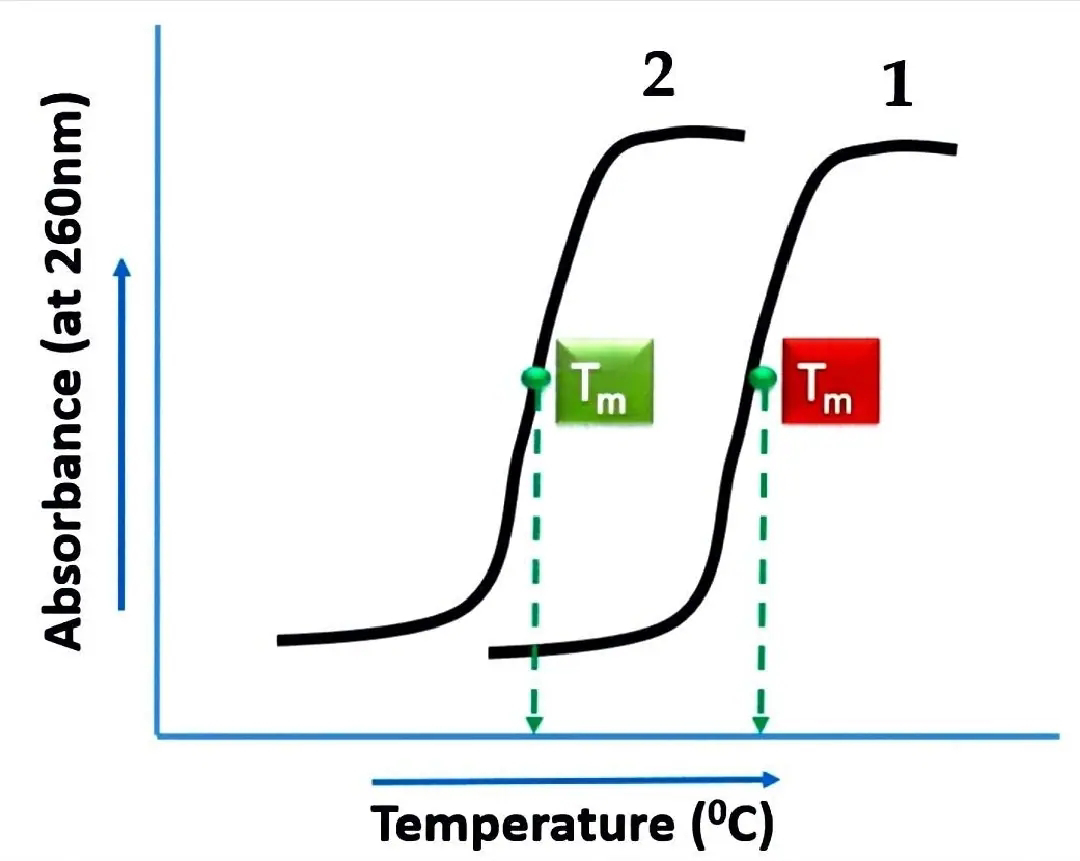# Factors Influencing Melting Temperature of DNA

The melting temperature of the DNA is the temperature at which half of the DNA molecules are denatured.

That means at this temperature half of the DNA molecules present in the solution will be single-stranded and other half will be double-stranded. melting temperature is found at the midpoint of the melting curve besides denaturation.

## Factors Affecting Melting Temperature

The melting temperature of DNA is affected by three main factors these are
• Nucleotide content of the DNA molecule
• Length of the DNA molecule and
• Ionic strength of the DNA solution

### Nucleotide content of the DNA molecule

DNA is double helix molecule. In DNA molecule Adenine always pairs with Thymine by 2 hydrogen bonds and Guanine always pair with Cytosine by 3 hydrogen bonds. Moreover the base stacking interactions in case of Guanine, Cytosine pair are more stable.

DNA molecule which is GC-rich in other words major percentage of this DNA molecule is made up of Guanine and Cytosine pairs and in the other DNA molecule in which Guanine, Cytosine pairs are less a DNA molecule which is GC-rich DNA will have higher melting temperature

This is because more heat energy is required to disrupt the stable base stacking interaction in this molecule. Thus the melting temperature of DNA is influenced by it's GC content. This can also be shown graphically (figure).As per the above figure, you can see two melting curves. As we know higher the GC content of DNA, higher will be the melting temperature. So the first DNA sequence which is GC-rich and the second DNA sequence is not GC rich.

The melting temperature at first DNA sequence is higher than the second DNA sequence.

### Length of the DNA molecule

Second factor affecting the melting temperature of DNA is the lengths of the DNA molecule.

A longer molecule of double-stranded DNA requires more energy to get disrupted as compared to a shorter molecule. This is because longer the molecule greater the stabilizing forces between the two DNA strands more heat energy is required to dissociate the strands and hence higher will be the melting temperature.

### Ionic strength of the DNA solution

The third factor affecting melting temperature of DNA is the ionic strength of the DNA solution.

The backbone of a DNA helix is made up of sugar and Phosphate. Each phosphate group in a DNA strand carries a negative charge. Thus overall each strand of DNA molecule carries a negative charge. The negative charges on both DNA strands will repel each other.

In eukaryotic cells proteins known as histones play important role in compaction of DNA within the nucleus of the cell. Histone proteins are rich in basic amino acids their positive charge helped in neutralizing the negative charges on DNA molecule.

In the laboratory the DNA molecules present in a solution are stabilized by adding positively charged ions, such as sodium (Na+). Being positively charged these ions bind to the sugar phosphate backbone and neutralize the negative charges on the phosphate groups. Thus DNA in a solution becomes stable ionic strength.

Suppose we have same DNA molecules in the given two DNA solutions. But the sodium chloride added in the first solution is 50 milli molar and in the second solution sodium chloride added is 100 millimolar.

This means ionic strength in second solution is more than the first solution. In other words more sodium ions are present in the second solution is compared to the first.
So in the second solution where ionic strength is high DNA molecule will be more stable and we will require more heat energy to denature these DNA molecules.
But in the first solution since ionic strength is low DNA molecules will be less stable as compared to the first this is because negative charges, which are not mute relized by sodium ions will repel each other.
This will contribute in the disruption of forces between the two strands. Therefore less heat energy will be required to denature these DNA molecules.
So we can conclude that higher the ionic strength of a DNA solution more heat energy required and higher will be the melting temperature similarly lower the ionic strength lower will be the melting temperature.